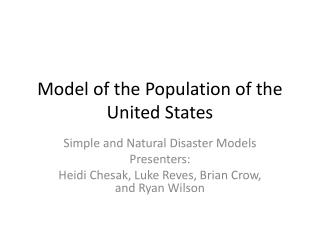DownloadDownload PresentationModel of the Population of the United States

# Model of the Population of the United States

Télécharger la présentation## Model of the Population of the United States

- - - - - - - - - - - - - - - - - - - - - - - - - - - E N D - - - - - - - - - - - - - - - - - - - - - - - - - - -
##### Presentation Transcript

1. Model of the Population of the United States Simple and Natural Disaster Models Presenters: Heidi Chesak, Luke Reves, Brian Crow, and Ryan Wilson

2. Growth rate population model. • The growth rate population model is • Where A is the growth coefficient, p0 is the initial population, p is the population at time t, and p1 is the population limit. • Here A=0.00012, p0=3.73 and p1 =251.7812.

3. Growth rate population model with natural disaster term • The growth rate population model is • Where A is the growth coefficient, p0 is the initial population, p is the population at time t, p1 is the population limit, and a is the natural disaster coefficient. • Here A=0.00012, p0=3.73 and p1 =251.7812.

4. Population model in 20 years. Note: Population is in millions. The population model in the first 20 years shows almost linear growth, topping the population around 7.2 million. The U.S. census data indicates that the population was 7.23 million. So the model is fairly close to the actual data with initial data values.

5. Population model in the long term To observe the long term behavior of the population model, the time of t was set to 220 years, which is the time since the U.S. census began. The model shows exponential growth, then to linear and then logarithmic growth. The growth appears to slow down at around 250 million.

6. U.S. Census graph This is a table plot from Scilab with values of the U.S. Census taken from http://www.census.gov/. The growth is entirely exponential, save for a few “hiccups”. The population since 2010 is 308 million people.

7. Population model vs. census data This is a side by side comparison to the population growth model and the U.S. census data set. For about the first 125 years, the model seems to be reasonably accurate, then the two start to drift apart. The population model seems to reach an equilibrium at p1=251 million.

8. Population growth with p1=308 To get a better understanding of the behavior of the model, we can change some of the variables and observe on the changes. This model has the limiting variable, p1 set to the current U.S. population of 308 million. The result is that the model and the actual growth reach similar ending points, but show vastly different growth rates.

9. Conclusions about the simple model. • The simple model is good for estimating the population growth for up to 120 years. • The p1 variable is equivalent to the equilibrium value of the growth model. • Since U.S. population growth is almost always exponential, the simple model can be used to projected population growth on short time scales.

10. Code for Simple Model • This is the code I used in scilab to graph the population growth by U.S. census data: • t=[0,10,20,30,40,50,60,70,80,90,100,110,120,130,140,150,160,170,180,190,200,210,220]; p=[3.93, 5.31, 7.24, 9.64,12.86,17.06, 23.19, 31.44,38.56,50.19,62.98,76.21,92.23,106.02,123.20,132.16,151.33,179.32, 203.30, 226.54, 248.71,281.42,308.74];plot(t,p,);for i=1:23,end;

11. Population Growth Behavior, 0 ≤T ≤20: Simple vs. Natural Disaster Simple Model Natural Disaster Model P(20) = 6.743 M P1(20) = 6.546 M

12. Population Long-Term Behavior, 0 ≤ T ≤ 400: Simple vs. Natural Disaster Simple Model Long Term Complex Model Long Term P(400) = 251.687 M P1(400) =239.198 M

13. A closer look at P1(400) Note that as the Population curve increases, a sinusoid reduces the rate of population growth with a period T=1 year. This affects the long-term behavior of the model as well, by both taking longer to reach equilibrium and by having a maximum population at equilibrium which will decrease with each disaster, again with a period of T=1 year. Also, as the population gets closer to Peq (Population at Equilibrium P1) the magnitude of natural disaster’s effect on population increases – i.e. the distance from peak to trough increases.

14. A Comparison with actual data As population neared equilibrium, the growth rate slowed, which is not reflected in the census data. This indicates that the model is effective up until approximately 1950, when U.S. Population growth entered a linear period. Data from U.S. Decennial Census obtained from www.census.gov